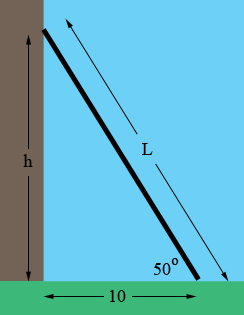SEARCH HOMEMath Central Quandaries & QueriesPlease, a student: A ladder makes an angle of 50 degrees with the ground. if the base of the ladder is 10 feet from the building, how high up the building does the ladder reach? i saw that someone else had a similar question, but we arn't using the pythagorean therom. we are using trig functions. Most of the problems we are doing is with the tangent. so this one probably is too.Hi,

I let L be the length of the ladder and h be the height the ladder reaches up the wall.From the diagram what is tan(50 degrees)? Solve for h.

Now a question from me. How long is the ladder?

PennyMath Central is supported by the University of Regina and The Pacific Institute for the Mathematical Sciences.